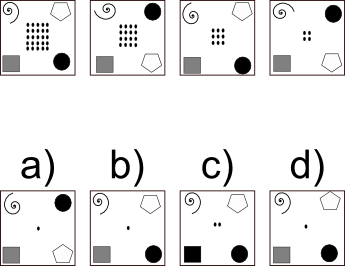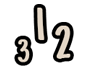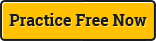QUESTION:

Which square comes next in the sequence?

We recommend practicing these tests under real timed test conditions. Take a free timed inductive test right now.

EXPLANATION:

The spiral and square rotate 90° clockwise each step. The circle and pentagon swap places with each other each step. The number of dots in the centre decreases by 1 square each time. I.e. the pattern is 5^2, 4^2, 3^2 etc.Numerical reasoning testsDiagrammatic reasoning testsVerbal reasoning tests